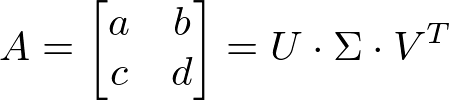# Singular value decomposition of a 2x2 matrix (C++ code)Dropping some [code here] to do a singular value decomposition (SVD) of a 2 by 2 real matrix.

Surprisingly there were not a lot of codes out there that satisfied me and were easily adaptable. So I decided to adapt some Eigen code for the specific case of a 2x2 matrix. It should not be difficult to change my types (matrix 2x2 and vector 2) to yours.

Usage:

```#include "svd_2x2.hpp"
Tbx::Mat2 M( a, b,
c, d);
// Compute full svd of M
Tbx::SVD_2x2 svd(M);

// Retrieve M = U * S * Vt
Tbx::Mat2 Vt = svd.matrix_v().transpose();
Tbx::Mat2 U = svd.matrix_u();
Tbx::Vec2 = svd.singular_values(); // Singular values diagonal matrix as a simple vector.
```